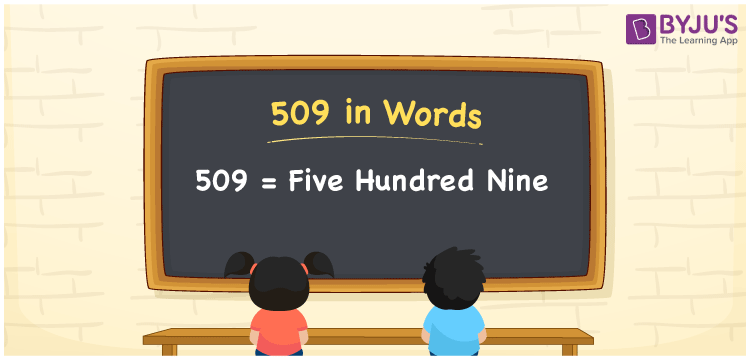# 509 in words

509 in words is written as Five Hundred and Nine. 509 represents the count or value. The article on Counting Numbers can give you an idea about count or counting. The number 509 is a 3 digit number that is used in expressions related to money, days, distance, length, weight and so on. Let us consider an example for 509. “The apples weighed 9 grams more than half a kg. It means that the apples weighed Five Hundred and Nine grams.”

 509 in words Five Hundred and Nine Five Hundred and Nine in Numbers 509

## 509 in English Words## How to Write 509 in Words?

We can convert 509 to words using a place value chart. The number 509 has 3 digits, so let’s make a chart that shows the place value up to 3 digits.

 Hundreds Tens Ones 5 0 9

Thus, we can write the expanded form as:

5 × Hundred + 0 × Ten + 9 × One

= 5 × 100 + 0 × 10 + 9 × 1

= 509

= Five Hundred and Nine.

509 is the natural number that is succeeded by 508 and preceded by 510.

509 in words – Five Hundred and Nine.

Is 509 an odd number? – Yes.

Is 509 an even number? – No.

Is 509 a perfect square number? – No.

Is 509 a perfect cube number? – No.

Is 509 a prime number? – Yes.

Is 509 a composite number? – No.

## Solved Example

1. Write the number 509 in expanded form

Solution: 5 × 100 + 0 × 10 + 9 × 1

We can write 509 = 500 + 00 + 9

= 5 × 100 + 0 × 10 + 9 × 1.

## Frequently Asked Questions on 509 in words

Q1

### How to write the number 509 in words?

509 in words is written as Five Hundred and Nine.
Q2

### Is 509 divisible by 3?

No. 509 is not divisible by 3.
Q3

### Is 509 a prime number?

Yes. 509 is a prime number.